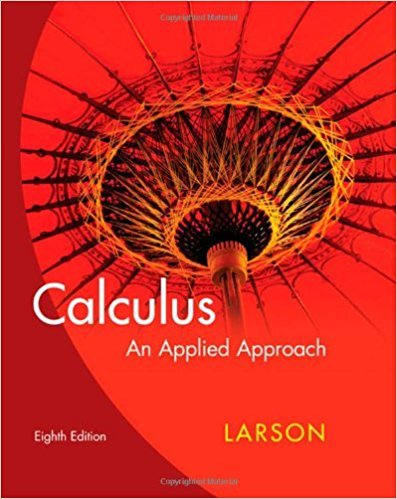×
×

# Solutions for Chapter 2: Differentiation## Full solutions for Calculus: An Applied Approach | 8th Edition

ISBN: 9780618958252Solutions for Chapter 2: Differentiation

Solutions for Chapter 2
4 5 0 394 Reviews
12
4
##### ISBN: 9780618958252

This expansive textbook survival guide covers the following chapters and their solutions. Calculus: An Applied Approach was written by and is associated to the ISBN: 9780618958252. This textbook survival guide was created for the textbook: Calculus: An Applied Approach , edition: 8. Since 100 problems in chapter 2: Differentiation have been answered, more than 21913 students have viewed full step-by-step solutions from this chapter. Chapter 2: Differentiation includes 100 full step-by-step solutions.

Key Calculus Terms and definitions covered in this textbook
• Absolute minimum

A value ƒ(c) is an absolute minimum value of ƒ if ƒ(c) ? ƒ(x)for all x in the domain of ƒ.

• Average velocity

The change in position divided by the change in time.

• Control

The principle of experimental design that makes it possible to rule out other factors when making inferences about a particular explanatory variable

• Difference of complex numbers

(a + bi) - (c + di) = (a - c) + (b - d)i

• Equilibrium point

A point where the supply curve and demand curve intersect. The corresponding price is the equilibrium price.

• Expanded form

The right side of u(v + w) = uv + uw.

• Function

A relation that associates each value in the domain with exactly one value in the range.

• Hypotenuse

Side opposite the right angle in a right triangle.

• Imaginary axis

See Complex plane.

• Inequality

A statement that compares two quantities using an inequality symbol

• Inverse relation (of the relation R)

A relation that consists of all ordered pairs b, a for which a, b belongs to R.

• Logarithmic form

An equation written with logarithms instead of exponents

• Lower bound test for real zeros

A test for finding a lower bound for the real zeros of a polynomial

• Mapping

A function viewed as a mapping of the elements of the domain onto the elements of the range

• Present value of an annuity T

he net amount of your money put into an annuity.

An angle in standard position whose terminal side lies on an axis.

• Reference triangle

For an angle ? in standard position, a reference triangle is a triangle formed by the terminal side of angle ?, the x-axis, and a perpendicular dropped from a point on the terminal side to the x-axis. The angle in a reference triangle at the origin is the reference angle

• Sphere

A set of points in Cartesian space equally distant from a fixed point called the center.

• Sum of two vectors

<u1, u2> + <v1, v2> = <u1 + v1, u2 + v2> <u1 + v1, u2 + v2, u3 + v3>

• Tree diagram

A visualization of the Multiplication Principle of Probability.

×